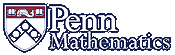Math 425: Partial Differential Equations### SOME REFERENCES

#### Our Text:

• Weinberger, H. L. A First Course in Partial Differential Equations: with Complex Variables and Transform Methods, Dover paperback (paperback reprinted from the 1965 edition); ISBN-13: 978-0486686400

#### Alternate Text:

• Strauss, Walter A., Partial differential equations: An Introduction, New York: Wiley, 1992. [Often used as a text for Math 425]

#### Background, with physical motivation and some applications

• Tikhonov and Samarskii, Equations of Mathematical Physics, translated from the Russian, Pergamon Press, 1963. Reprinted by Dover Publications, 1990.

#### Basic general texts: beginning graduate level

• Carrier, G. F. and C. E. Pearson, Partial Differential Equations, Academic Press, 1976; Second edition, 1988. [Less theoretical, aimed at people trying to solve practical problems.]

• DiBenedetto, E., Partial Differential Equations, Birkhauser, 1995.

• Evans, Lawrence C., Partial Differential Equations, in the series Graduate studies in mathematics, v. 19, American Math. Society, Second Edition, 2010.

• John, F., Partial Differential Equations, 4th edition, Springer Applied Mathematics Series, 1982.

• McOwen, R., Partial Differential Equations: Methods and Applications, Prentice-Hall, 1996

• Renardy, Michael and Rogers, Robert C., An Introduction to Partial Differential Equations, New York: Springer-Verlag, 1993, Texts in Applied Mathematics; 13.

• JLK Australia 2008 Notes